Question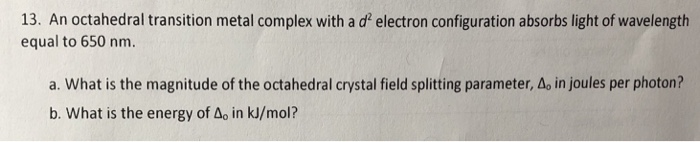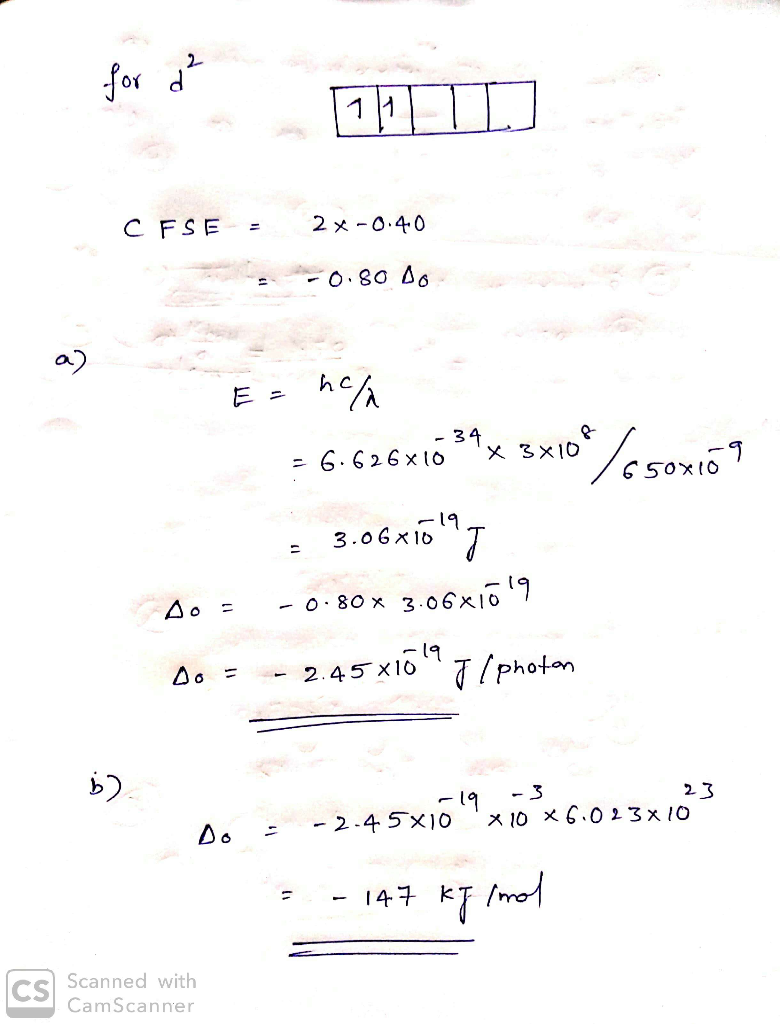#### Earn Coins

Coins can be redeemed for fabulous gifts.

Similar Homework Help Questions
• ### An octahedral metal complex absorbs light with wavelength 580 nm. a. What is the crystal field...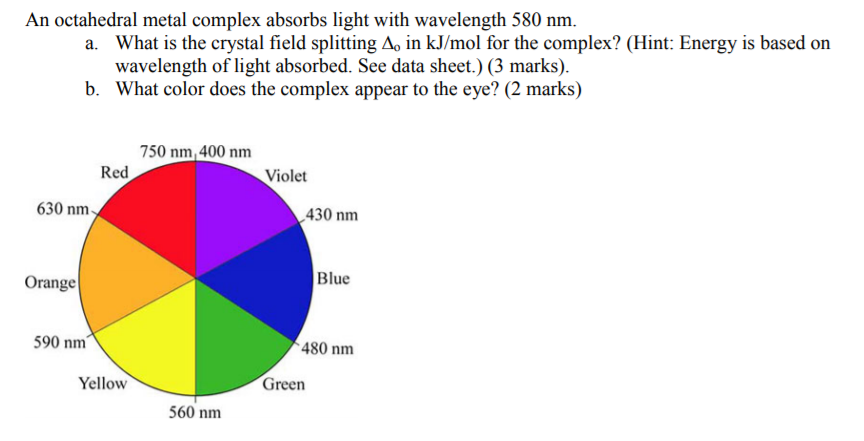An octahedral metal complex absorbs light with wavelength 580 nm. a. What is the crystal field splitting A, in kJ/mol for the complex? (Hint: Energy is based on wavelength of light absorbed. See data sheet.) (3 marks) b. What color does the complex appear to the eye? (2 marks) 750 nm, 400 nm Red Violet 630 nm 430 nm Blue Orange 590 nm 480 nm Yellow Green 560 nm

• ### A ?1 octahedral complex is found to absorb visible light, with the absorption maximum occurring at 499 nm . Calculate the crystal-field splitting energy, Δ , in kJ/mol.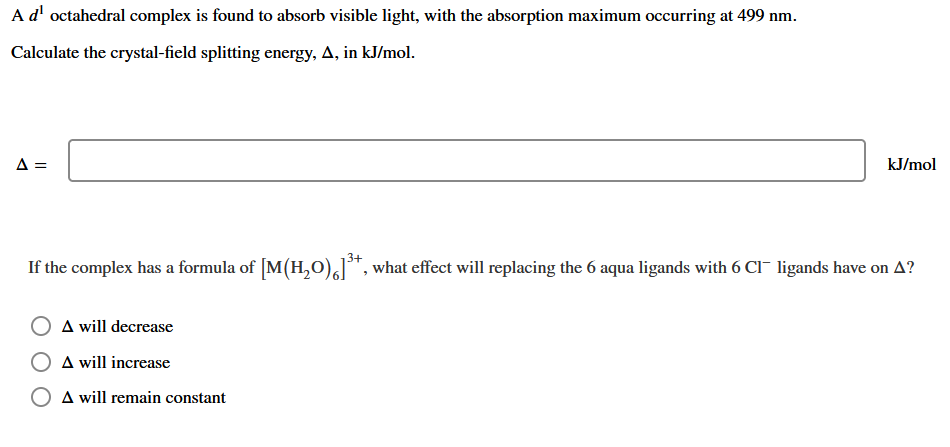A ?1 octahedral complex is found to absorb visible light, with the absorption maximum occurring at 499 nmCalculate the crystal-field splitting energy, Δin kJ/mol.

• ### The extent of crystal field splitting is often determined from spectra. Given the wavelength (7.) of...The extent of crystal field splitting is often determined from spectra. Given the wavelength (7.) of maximum absorption, 2. = 295 nm, find the crystal field splitting energy (A), in kJ/mol, for the complex ion (Rh(NH3).13+. kJ/mol

• ### Ad octahedral complex is found to absorb visible light, with the absorption maximum occurring at 515...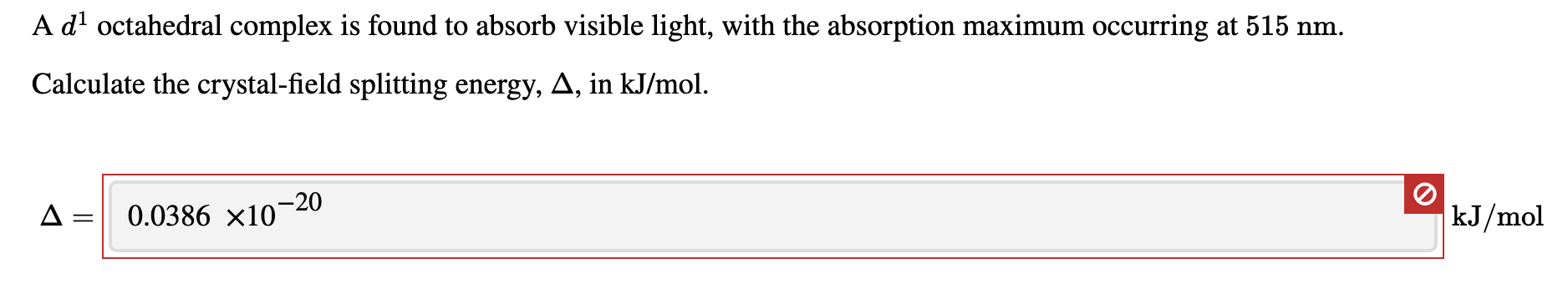Ad octahedral complex is found to absorb visible light, with the absorption maximum occurring at 515 nm. Calculate the crystal-field splitting energy, A, in kJ/mol. A = 0.0386 x10-20 kJ/mol

• ### The wavelength of light (in nanometers) that is absorbed by a complex that has a crystal-field...

The wavelength of light (in nanometers) that is absorbed by a complex that has a crystal-field splitting energy of 3.30×102 kJ/mol is _____. (h = 6.626 × 10−34 J·s, c = 3.00 × 108 m/s)

• ### B3. An octahedral transition metal complex with the structure shown below was isolated from the reaction...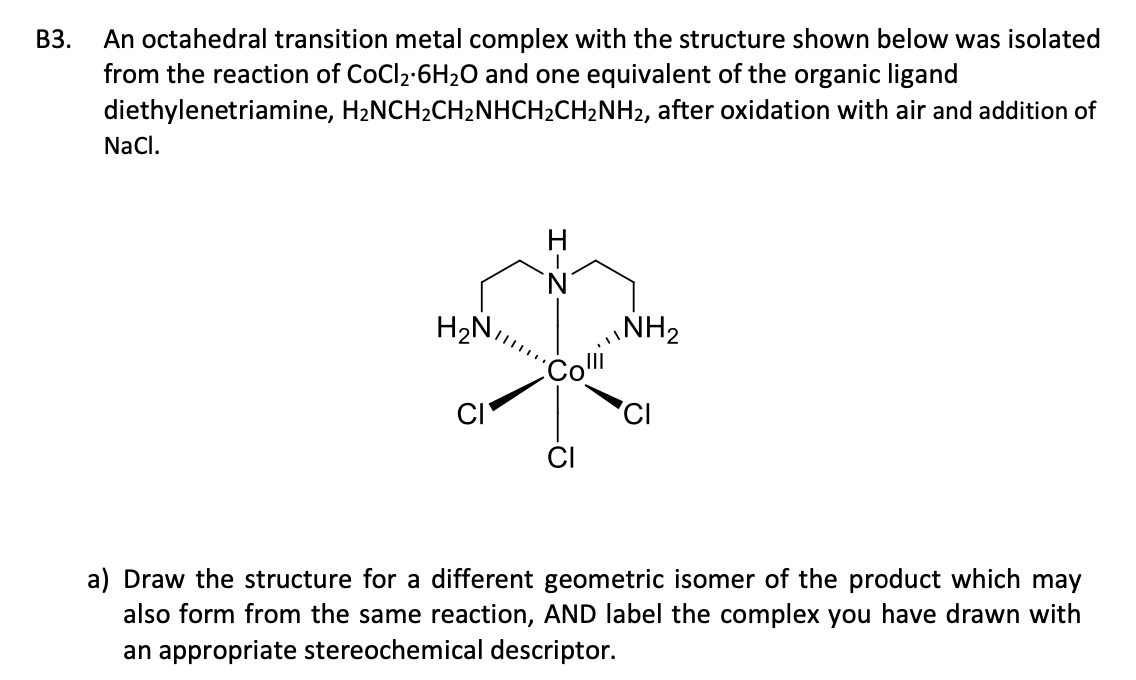B3. An octahedral transition metal complex with the structure shown below was isolated from the reaction of CoCl2.6H20 and one equivalent of the organic ligand diethylenetriamine, H2NCH2CH2NHCH2CH2NH2, after oxidation with air and addition of Naci. I-Z H2N,,, NH2 com CICI a) Draw the structure for a different geometric isomer of the product which may also form from the same reaction, AND label the complex you have drawn with an appropriate stereochemical descriptor. The complex shown on the previous page is...

• ### Estimate the crystal field stabilization energy for the octahedral ion hexacyanocobaltate(III), if the wavelength of maximum...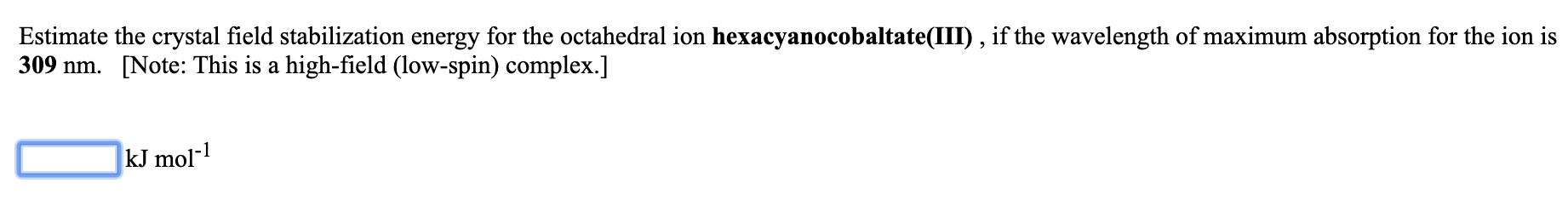Estimate the crystal field stabilization energy for the octahedral ion hexacyanocobaltate(III), if the wavelength of maximum absorption for the ion is 309 nm. [Note: This is a high-field (low-spin) complex.] kJ mol-1

• ### Estimate the crystal field stabilization energy for the octahedral ion hexafluorocobaltate(III), if the wavelength of maximum...

Estimate the crystal field stabilization energy for the octahedral ion hexafluorocobaltate(III), if the wavelength of maximum absorption for the ion is 710 nm.   [Note: This is a weak-field (high-spin) complex.] _____ kJ mol-1

• ### A dl octahedral complex is found to absorb visible light, with the absorption maximum occurring at...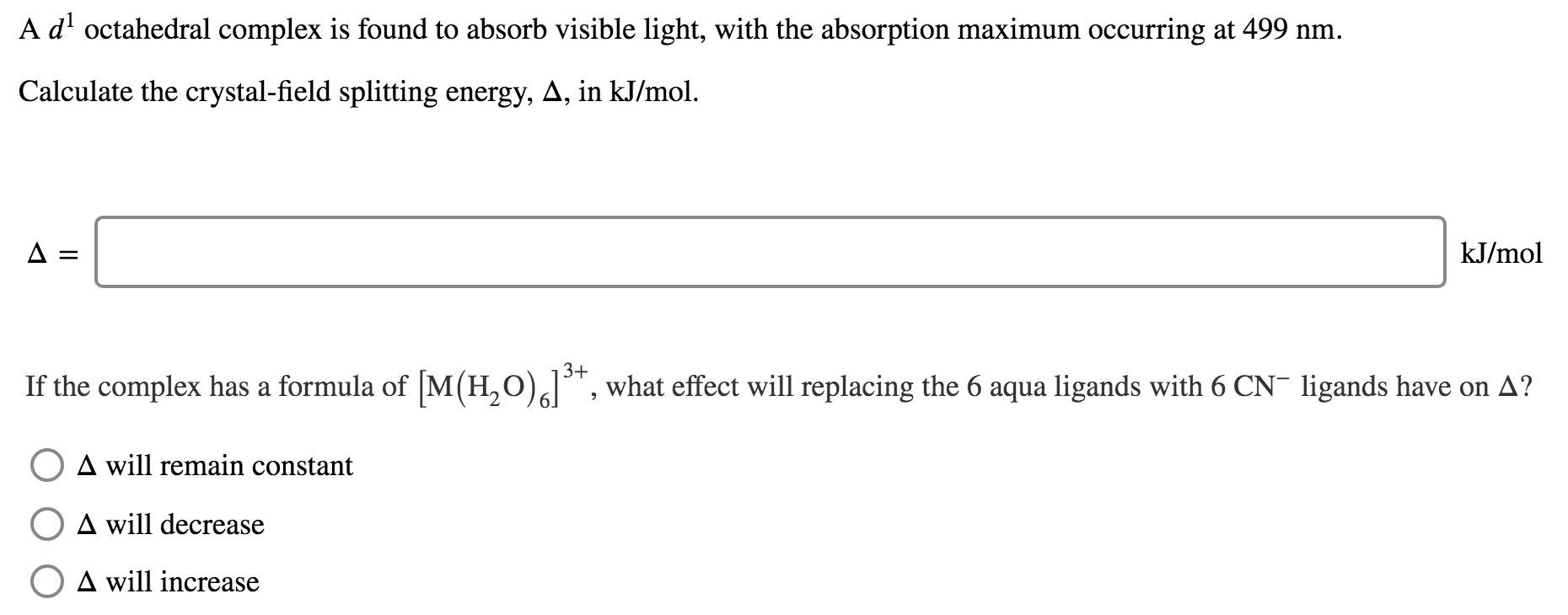A dl octahedral complex is found to absorb visible light, with the absorption maximum occurring at 499 nm. Calculate the crystal-field splitting energy, A, in kJ/mol. A= kJ/mol If the complex has a formula of [M(H20)alt, what effect will replacing the 6 aqua ligands with 6 CN- ligands have on A? O A will remain constant A will decrease O A will increase

• ### For an octahedral transition metal complex, a stronger ligand field Select one or more: O results...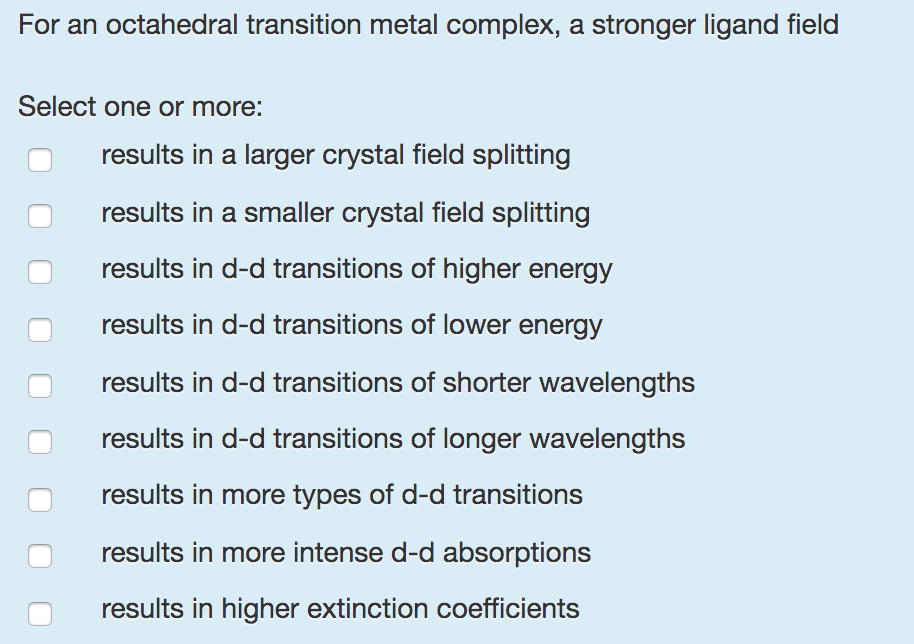For an octahedral transition metal complex, a stronger ligand field Select one or more: O results in a larger crystal field splitting results in a smaller crystal field splitting results in d-d transitions of higher energy results in d-d transitions of lower energy results in d-d transitions of shorter wavelengths results in d-d transitions of longer wavelengths results in more types of d-d transitions results in more intense d-d absorptions results in higher extinction coefficients